Visualization Example: Polyhedra

We now demonstrate the use of matrix multiplication for manipulating an object, specifically an octohedron. The Octahedron is made up of eight polygons and the initial coordinates of the vertices were set to make a regular octahedron with its main diagonals parallel to axes x,y,z. The faces of the octahedron are colored so that rotations and other transformations can be easily tracked.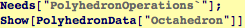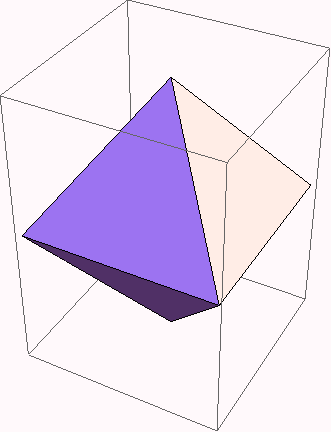Above, the color of the three dimensional object derives from the colors in the light sources. For example, note that there appears to be a blue light pointing down from the left. The lights stay fixed as we rotate the object. If Lighting → None, then the polyhedron's faces will appear to be black.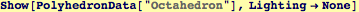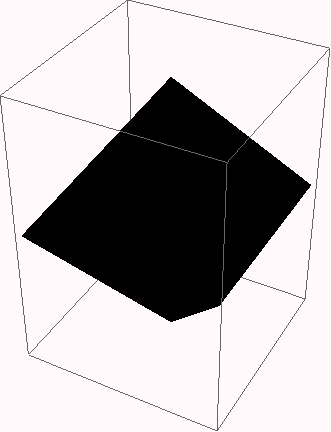We can extract data from the Octahedron, and build our own with individually colored faces. We will need the individual colors to identify what happens to the polyhedron under linear transformaions.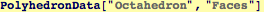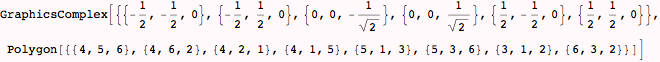The object ColOct is defined below to draw an octahedron and it invokes the Polygon function to draw the triangular faces by connecting three points at specific numerical coordinates that we obtain from the Octahedron data. Because we will turn off lighting, we will ask that each of the faces glow, using the Glow graphics directive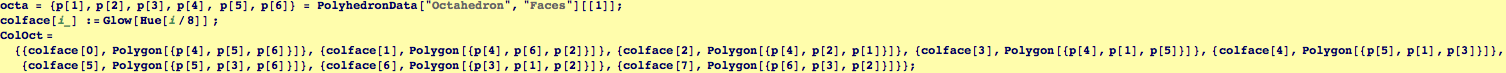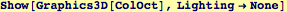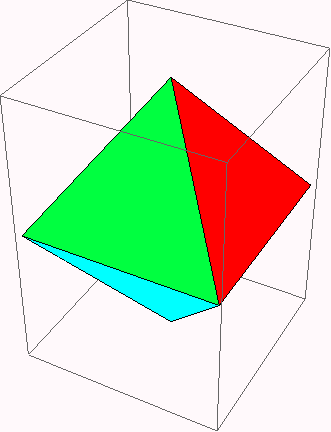Created by Wolfram Mathematica 6.0  (06 September 2007)